Скачать презентацию Pressure Drop Through an Orifice as Measured by

860cc9544473ae0b99d855e81a1c07ae.ppt

• Количество слайдов: 10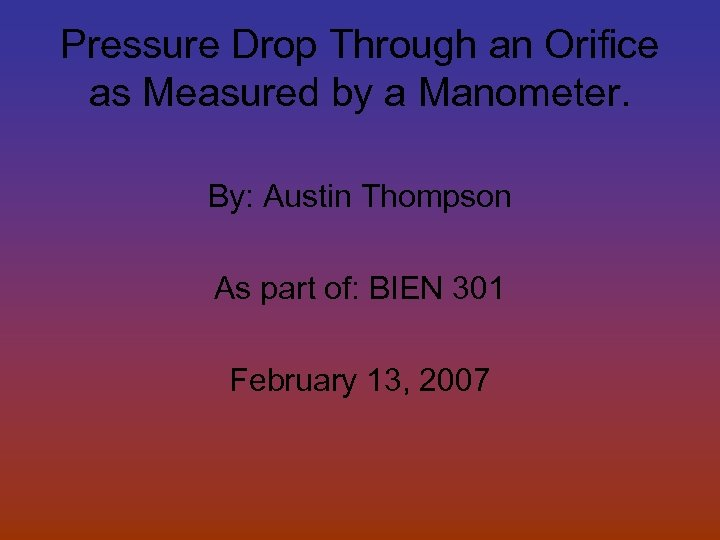Pressure Drop Through an Orifice as Measured by a Manometer. By: Austin Thompson As part of: BIEN 301 February 13, 2007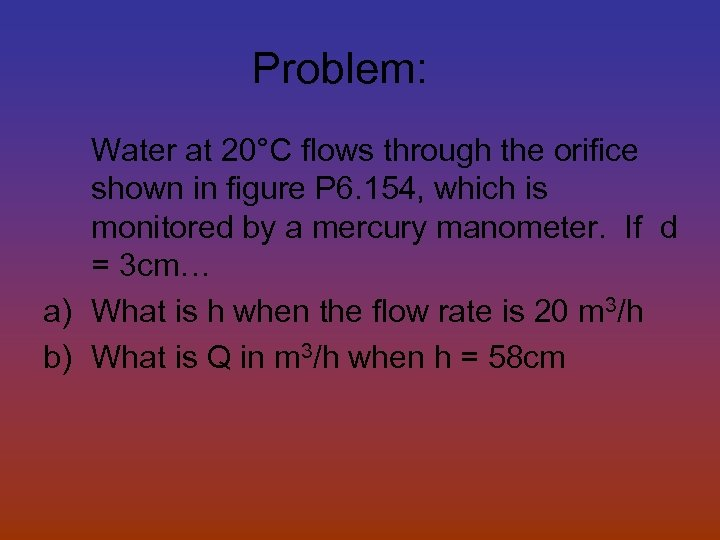Problem: Water at 20°C flows through the orifice shown in figure P 6. 154, which is monitored by a mercury manometer. If d = 3 cm… a) What is h when the flow rate is 20 m 3/h b) What is Q in m 3/h when h = 58 cm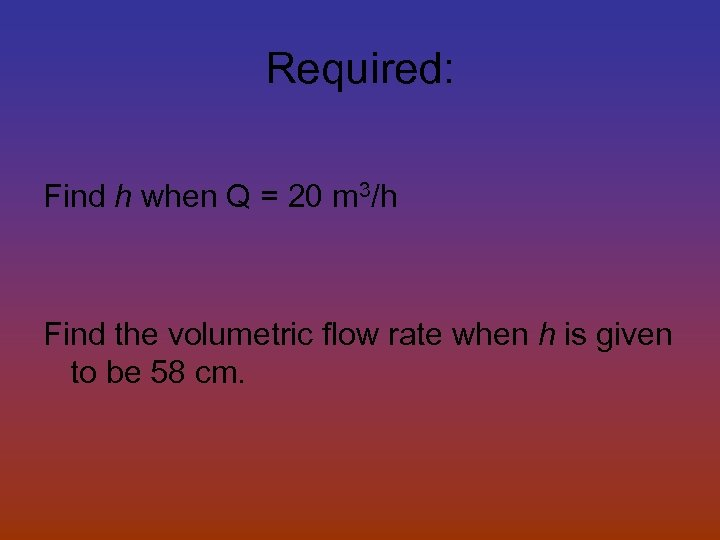Required: Find h when Q = 20 m 3/h Find the volumetric flow rate when h is given to be 58 cm.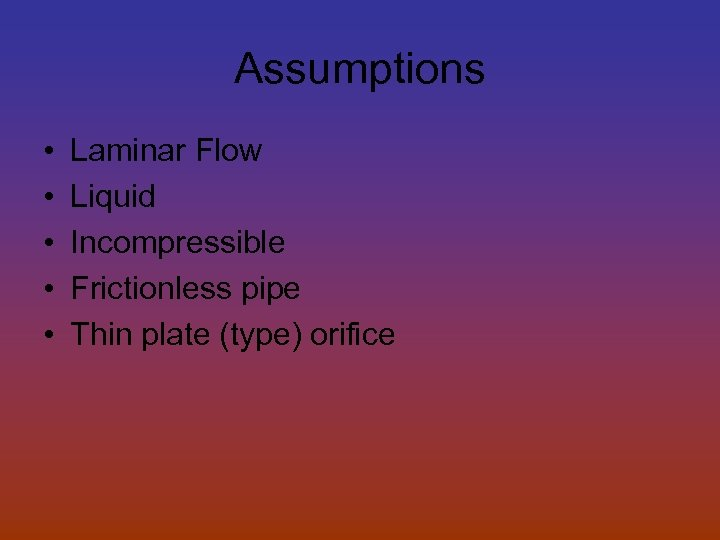Assumptions • • • Laminar Flow Liquid Incompressible Frictionless pipe Thin plate (type) orifice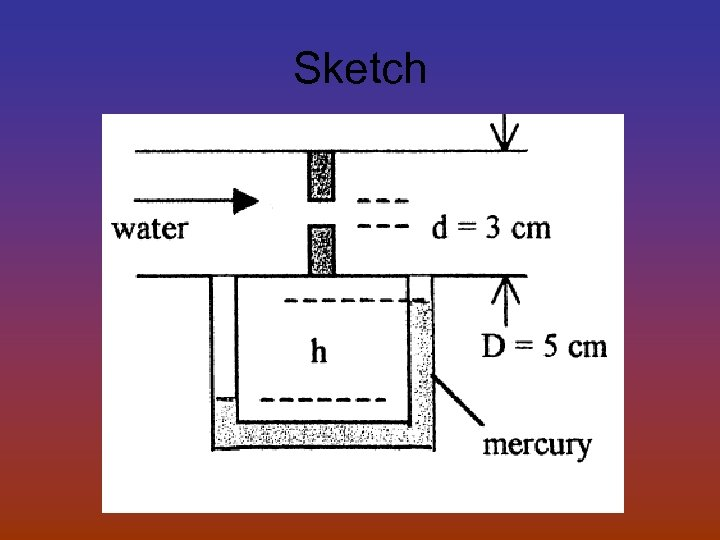Sketch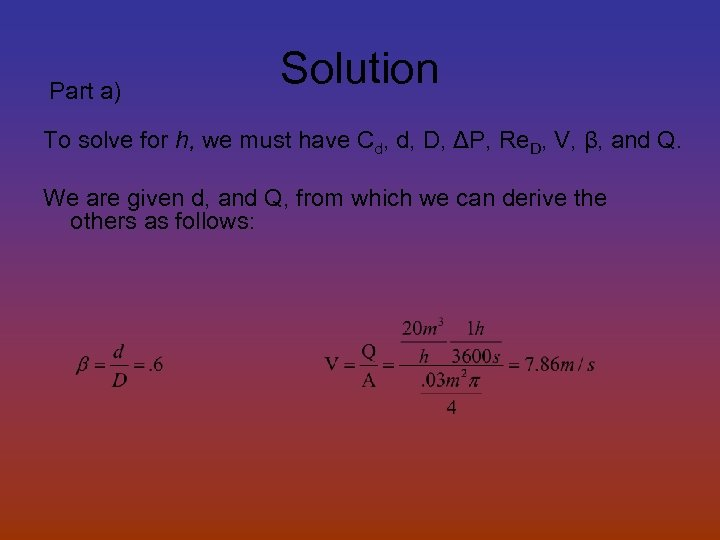Part a) Solution To solve for h, we must have Cd, d, D, ΔP, Re. D, V, β, and Q. We are given d, and Q, from which we can derive the others as follows:Now for the Reynolds Number: With the Reynolds Number now solved for, we can go to figure 6. 41 and find that Cd is about 0. 605.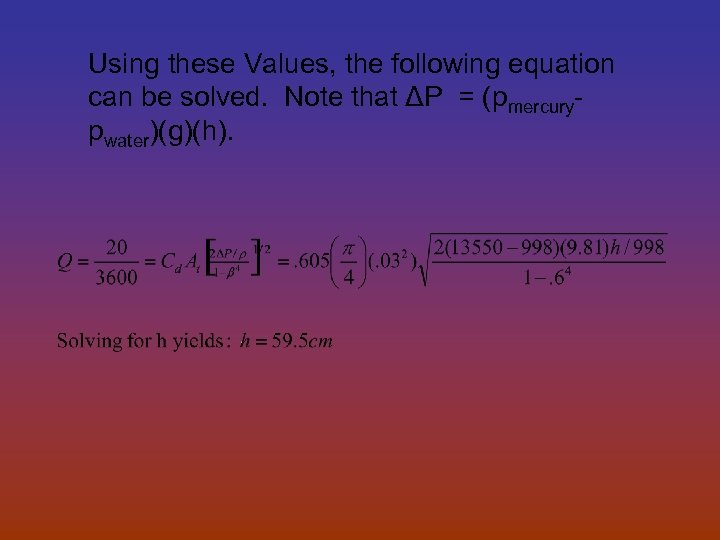Using these Values, the following equation can be solved. Note that ΔP = (pmercurypwater)(g)(h).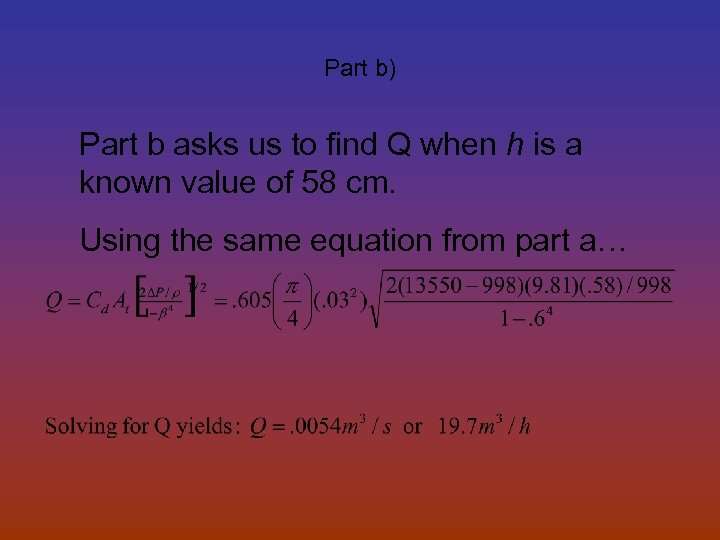Part b) Part b asks us to find Q when h is a known value of 58 cm. Using the same equation from part a…Biomedical Application This problem shows relationship in pressures across an orifice. Given that this example does have perfectly rounded corners and given dimensions, it could be compared to something in the body. A blood clot could be described as an orifice, only with more complex forms of exemplification. Knowing how to calculate pressure changes in an artery or vein may be vital knowledge in saving someone’s life.